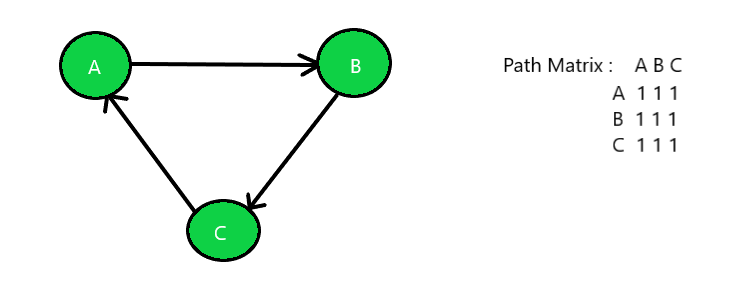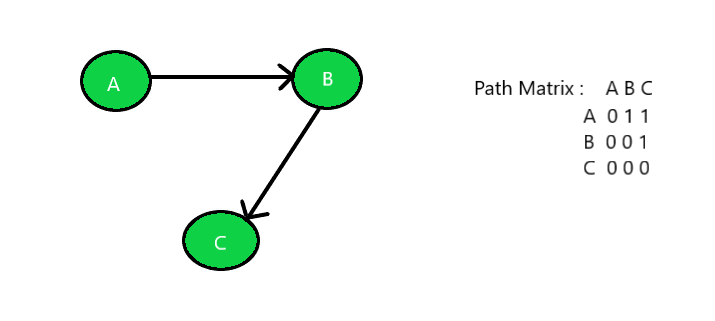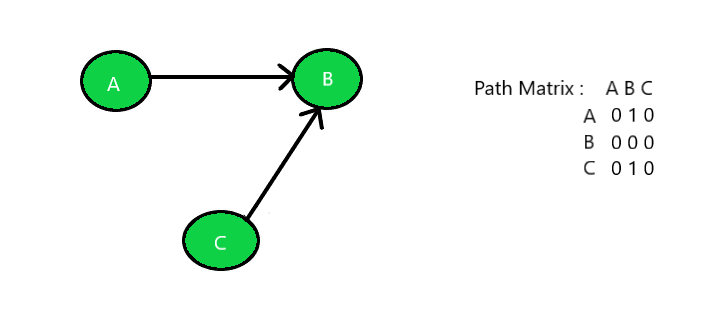Related Articles

# Check if a graph is Strongly, Unilaterally or Weakly connected

• Last Updated : 29 May, 2021

Given an unweighted directed graph G as a path matrix, the task is to find out if the graph is Strongly Connected or Unilaterally Connected or Weakly Connected.

Strongly Connected: A graph is said to be strongly connected if every pair of vertices(u, v) in the graph contains a path between each other. In an unweighted directed graph G, every pair of vertices u and v should have a path in each direction between them i.e., bidirectional path. The elements of the path matrix of such a graph will contain all 1’s.
Unilaterally Connected: A graph is said to be unilaterally connected if it contains a directed path from u to v OR a directed path from v to u for every pair of vertices u, v. Hence, at least for any pair of vertices, one vertex should be reachable form the other. Such a path matrix would rather have upper triangle elements containing 1’s OR lower triangle elements containing 1’s.
Weakly Connected: A graph is said to be weakly connected if there doesn’t exist any path between any two pairs of vertices. Hence, if a graph G doesn’t contain a directed path (from u to v or from v to u for every pair of vertices u, v) then it is weakly connected. The elements of such a path matrix of this graph would be random.

Examples:

Input: Below is the given graph with path matrix:Output: Strongly Connected Graph
Input: Below is the given graph with path matrix:Output: Unilaterally Connected Graph
Input: Below is the given graph with path matrix:Output: Weakly Connected Graph

Approach:

1. For the graph to be Strongly Connected, traverse the given path matrix using the approach discussed in this article check whether all the values in the cell are 1 or not. If yes then print “Strongly Connected Graph” else check for the other two graphs.
2. For the graph to be Unilaterally Connected, traverse the given path matrix using the approach discussed in this article and check the following:
• If all the values above the main diagonal are 1s and all the values other than that are 0s.
• If all the values below the main diagonal are 1s and all the values other than that are 0s.
3. If one of the above two conditions satisfies then the given graph is Unilaterally Connected else the graph is Weakly Connected Graph.

Below is the implementation of the above approach:

## C++

 `// C++ implementation of the approach` `#include ``using` `namespace` `std;``#define V 3` `// Function to find the characteristic``// of the given graph``int` `checkConnected(``int` `graph[][V], ``int` `n)``{` `    ``// Check whether the graph is``    ``// strongly connected or not``    ``bool` `strongly = ``true``;` `    ``// Traverse the path matrix``    ``for` `(``int` `i = 0; i < n; i++) {` `        ``for` `(``int` `j = 0; j < n; j++) {` `            ``// If all the elements are``            ``// not equal then the graph``            ``// is not strongly connected``            ``if` `(graph[i][j] != graph[j][i]) {``                ``strongly = ``false``;``                ``break``;``            ``}``        ``}` `        ``// Break out of the loop if false``        ``if` `(!strongly) {``            ``break``;``        ``}``    ``}``    ``// If true then print strongly``    ``// connected and return``    ``if` `(strongly) {``        ``cout << ``"Strongly Connected"``;``        ``return` `0;``    ``}` `    ``// Check whether the graph is``    ``// Unilaterally connected by``    ``// checking Upper Triangle element``    ``bool` `uppertri = ``true``;` `    ``// Traverse the path matrix``    ``for` `(``int` `i = 0; i < n; i++) {` `        ``for` `(``int` `j = 0; j < n; j++) {` `            ``// If uppertriangle elements``            ``// are 0 then break out of the``            ``// loop and check the elements``            ``// of lowertriangle matrix``            ``if` `(i > j && graph[i][j] == 0) {``                ``uppertri = ``false``;``                ``break``;``            ``}``        ``}` `        ``// Break out of the loop if false``        ``if` `(!uppertri) {``            ``break``;``        ``}``    ``}` `    ``// If true then print unilaterally``    ``// connected and return``    ``if` `(uppertri) {``        ``cout << ``"Unilaterally Connected"``;``        ``return` `0;``    ``}` `    ``// Check lowertraingle elements``    ``bool` `lowertri = ``true``;` `    ``// Traverse the path matrix``    ``for` `(``int` `i = 0; i < n; i++) {` `        ``for` `(``int` `j = 0; j < n; j++) {` `            ``// If lowertraingle elements``            ``// are 0 then break cause``            ``// 1's are expected``            ``if` `(i < j && graph[i][j] == 0) {``                ``lowertri = ``false``;``                ``break``;``            ``}``        ``}` `        ``// Break out of the loop if false``        ``if` `(!lowertri) {``            ``break``;``        ``}``    ``}` `    ``// If true then print unilaterally``    ``// connected and return``    ``if` `(lowertri) {``        ``cout << ``"Unilaterally Connected"``;``        ``return` `0;``    ``}` `    ``// If elements are in random order``    ``// unsynchronized then print weakly``    ``// connected and return``    ``else` `{``        ``cout << ``"Weakly Connected"``;``    ``}` `    ``return` `0;``}` `// Driver Code``int` `main()``{``    ``// Number of nodes``    ``int` `n = 3;` `    ``// Given Path Matrix``    ``int` `graph[V][V] = {``        ``{ 0, 1, 1 },``        ``{ 0, 0, 1 },``        ``{ 0, 0, 0 },``    ``};` `    ``// Function Call``    ``checkConnected(graph, n);``    ``return` `0;``}`

## Java

 `// Java implementation of the above approach``import` `java.util.*;` `class` `GFG{``    ` `static` `final` `int` `V = ``3``;` `// Function to find the characteristic``// of the given graph``static` `int` `checkConnected(``int` `graph[][], ``int` `n)``{``    ` `    ``// Check whether the graph is``    ``// strongly connected or not``    ``boolean` `strongly = ``true``;` `    ``// Traverse the path matrix``    ``for``(``int` `i = ``0``; i < n; i++)``    ``{``        ``for``(``int` `j = ``0``; j < n; j++)``        ``{``            ` `            ``// If all the elements are``            ``// not equal then the graph``            ``// is not strongly connected``            ``if` `(graph[i][j] != graph[j][i])``            ``{``                ``strongly = ``false``;``                ``break``;``            ``}``        ``}``        ` `        ``// Break out of the loop if false``        ``if` `(!strongly)``        ``{``            ``break``;``        ``}``    ``}``    ` `    ``// If true then print strongly``    ``// connected and return``    ``if` `(strongly)``    ``{``        ``System.out.print(``"Strongly Connected"``);``        ``return` `0``;``    ``}` `    ``// Check whether the graph is``    ``// Unilaterally connected by``    ``// checking Upper Triangle element``    ``boolean` `uppertri = ``true``;` `    ``// Traverse the path matrix``    ``for``(``int` `i = ``0``; i < n; i++)``    ``{``        ``for``(``int` `j = ``0``; j < n; j++)``        ``{``            ` `            ``// If uppertriangle elements``            ``// are 0 then break out of the``            ``// loop and check the elements``            ``// of lowertriangle matrix``            ``if` `(i > j && graph[i][j] == ``0``)``            ``{``                ``uppertri = ``false``;``                ``break``;``            ``}``        ``}` `        ``// Break out of the loop if false``        ``if` `(!uppertri)``        ``{``            ``break``;``        ``}``    ``}` `    ``// If true then print unilaterally``    ``// connected and return``    ``if` `(uppertri)``    ``{``        ``System.out.print(``"Unilaterally Connected"``);``        ``return` `0``;``    ``}` `    ``// Check lowertraingle elements``    ``boolean` `lowertri = ``true``;` `    ``// Traverse the path matrix``    ``for``(``int` `i = ``0``; i < n; i++)``    ``{``        ``for``(``int` `j = ``0``; j < n; j++)``        ``{``            ` `            ``// If lowertraingle elements``            ``// are 0 then break cause``            ``// 1's are expected``            ``if` `(i < j && graph[i][j] == ``0``)``            ``{``                ``lowertri = ``false``;``                ``break``;``            ``}``        ``}``        ` `        ``// Break out of the loop if false``        ``if` `(!lowertri)``        ``{``            ``break``;``        ``}``    ``}` `    ``// If true then print unilaterally``    ``// connected and return``    ``if` `(lowertri)``    ``{``        ``System.out.print(``"Unilaterally Connected"``);``        ``return` `0``;``    ``}` `    ``// If elements are in random order``    ``// unsynchronized then print weakly``    ``// connected and return``    ``else``    ``{``        ``System.out.print(``"Weakly Connected"``);``    ``}``    ``return` `0``;``}` `// Driver Code``public` `static` `void` `main(String[] args)``{``    ` `    ``// Number of nodes``    ``int` `n = ``3``;` `    ``// Given Path Matrix``    ``int` `graph[][] = { { ``0``, ``1``, ``1` `},``                      ``{ ``0``, ``0``, ``1` `},``                      ``{ ``0``, ``0``, ``0` `} };``                        ` `    ``// Function call``    ``checkConnected(graph, n);``}``}` `// This code is contributed by 29AjayKumar`

## Python3

 `# Python3 implementation of``# the above approach``V ``=` `3` `# Function to find the``# characteristic of the``# given graph``def` `checkConnected(graph, n):`` ` `    ``# Check whether the graph is``    ``# strongly connected or not``    ``strongly ``=` `True``;`` ` `    ``# Traverse the path``    ``# matrix``    ``for` `i ``in` `range``(n):``        ``for` `j ``in` `range``(n):`` ` `            ``# If all the elements are``            ``# not equal then the graph``            ``# is not strongly connected``            ``if` `(graph[i][j] !``=` `graph[j][i]):``                ``strongly ``=` `False``;``                ``break`` ` `        ``# Break out of the``        ``# loop if false``        ``if` `not` `strongly:``           ``break``;``        ` `    ``# If true then print``    ``# strongly connected and return``    ``if` `(strongly):``        ``print``(``"Strongly Connected"``);``        ``exit()   `` ` `    ``# Check whether the graph is``    ``# Unilaterally connected by``    ``# checking Upper Triangle element``    ``uppertri ``=` `True``;`` ` `    ``# Traverse the path matrix``    ``for` `i ``in` `range``(n):``        ``for` `j ``in` `range``(n):`` ` `            ``# If uppertriangle elements``            ``# are 0 then break out of the``            ``# loop and check the elements``            ``# of lowertriangle matrix``            ``if` `(i > j ``and` `graph[i][j] ``=``=` `0``):``                ``uppertri ``=` `False``;``                ``break``;            `` ` `        ``# Break out of the``        ``# loop if false``        ``if` `not` `uppertri:``            ``break``;    `` ` `    ``# If true then print``    ``# unilaterally connected``    ``# and return``    ``if` `uppertri:``        ``print``(``"Unilaterally Connected"``);``        ``exit()`` ` `    ``# Check lowertraingle elements``    ``lowertri ``=` `True``;`` ` `    ``# Traverse the path matrix``    ``for` `i ``in` `range``(n):``        ``for` `j ``in` `range``(n):`` ` `            ``# If lowertraingle elements``            ``# are 0 then break cause``            ``# 1's are expected``            ``if` `(i < j ``and` `graph[i][j] ``=``=` `0``):``                ``lowertri ``=` `False``;``                ``break``;`` ` `        ``# Break out of the``        ``# loop if false``        ``if` `not` `lowertri:``            ``break``;        `` ` `    ``# If true then print``    ``# unilaterally connected``    ``# and return``    ``if` `lowertri:``        ``print``(``"Unilaterally Connected"``)``        ``exit()`` ` `    ``# If elements are in random order``    ``# unsynchronized then print weakly``    ``# connected and return``    ``else``:``        ``print``(``"Weakly Connected"``)``    ` `    ``exit()` `if` `__name__ ``=``=` `"__main__"``:``    ` `    ``# Number of nodes``    ``n ``=` `3``;`` ` `    ``# Given Path Matrix``    ``graph ``=` `[[``0``, ``1``, ``1``],``             ``[``0``, ``0``, ``1``],``             ``[``0``, ``0``, ``0``]];`` ` `    ``# Function Call``    ``checkConnected(graph, n);``    ` ` ``# This code is contributed by rutvik_56`

## C#

 `// C# implementation of the above approach``using` `System;` `class` `GFG{``    ` `//static readonly int V = 3;` `// Function to find the characteristic``// of the given graph``static` `int` `checkConnected(``int` `[,]graph, ``int` `n)``{``    ` `    ``// Check whether the graph is``    ``// strongly connected or not``    ``bool` `strongly = ``true``;` `    ``// Traverse the path matrix``    ``for``(``int` `i = 0; i < n; i++)``    ``{``        ``for``(``int` `j = 0; j < n; j++)``        ``{``            ` `            ``// If all the elements are``            ``// not equal then the graph``            ``// is not strongly connected``            ``if` `(graph[i, j] != graph[j, i])``            ``{``                ``strongly = ``false``;``                ``break``;``            ``}``        ``}``        ` `        ``// Break out of the loop if false``        ``if` `(!strongly)``        ``{``            ``break``;``        ``}``    ``}``    ` `    ``// If true then print strongly``    ``// connected and return``    ``if` `(strongly)``    ``{``        ``Console.Write(``"Strongly Connected"``);``        ``return` `0;``    ``}` `    ``// Check whether the graph is``    ``// Unilaterally connected by``    ``// checking Upper Triangle element``    ``bool` `uppertri = ``true``;` `    ``// Traverse the path matrix``    ``for``(``int` `i = 0; i < n; i++)``    ``{``        ``for``(``int` `j = 0; j < n; j++) ``        ``{``            ` `            ``// If uppertriangle elements``            ``// are 0 then break out of the``            ``// loop and check the elements``            ``// of lowertriangle matrix``            ``if` `(i > j && graph[i, j] == 0)``            ``{``                ``uppertri = ``false``;``                ``break``;``            ``}``        ``}``        ` `        ``// Break out of the loop if false``        ``if` `(!uppertri)``        ``{``            ``break``;``        ``}``    ``}` `    ``// If true then print unilaterally``    ``// connected and return``    ``if` `(uppertri)``    ``{``        ``Console.Write(``"Unilaterally Connected"``);``        ``return` `0;``    ``}` `    ``// Check lowertraingle elements``    ``bool` `lowertri = ``true``;` `    ``// Traverse the path matrix``    ``for``(``int` `i = 0; i < n; i++)``    ``{``        ``for``(``int` `j = 0; j < n; j++)``        ``{``            ` `            ``// If lowertraingle elements``            ``// are 0 then break cause``            ``// 1's are expected``            ``if` `(i < j && graph[i, j] == 0)``            ``{``                ``lowertri = ``false``;``                ``break``;``            ``}``        ``}``        ` `        ``// Break out of the loop if false``        ``if` `(!lowertri)``        ``{``            ``break``;``        ``}``    ``}` `    ``// If true then print unilaterally``    ``// connected and return``    ``if` `(lowertri)``    ``{``        ``Console.Write(``"Unilaterally Connected"``);``        ``return` `0;``    ``}` `    ``// If elements are in random order``    ``// unsynchronized then print weakly``    ``// connected and return``    ``else``    ``{``        ``Console.Write(``"Weakly Connected"``);``    ``}``    ``return` `0;``}` `// Driver Code``public` `static` `void` `Main(String[] args)``{``    ` `    ``// Number of nodes``    ``int` `n = 3;` `    ``// Given Path Matrix``    ``int` `[,]graph = { { 0, 1, 1 },``                     ``{ 0, 0, 1 },``                     ``{ 0, 0, 0 } };``                        ` `    ``// Function call``    ``checkConnected(graph, n);``}``}` `// This code is contributed by 29AjayKumar`

## Javascript

 ``
Output:
`Unilaterally Connected`

Time Complexity: O(N2)
Auxiliary Space: O(1)

Attention reader! Don’t stop learning now. Get hold of all the important DSA concepts with the DSA Self Paced Course at a student-friendly price and become industry ready.  To complete your preparation from learning a language to DS Algo and many more,  please refer Complete Interview Preparation Course.

In case you wish to attend live classes with experts, please refer DSA Live Classes for Working Professionals and Competitive Programming Live for Students.

My Personal Notes arrow_drop_up